# Le Chatelier’s principle

## Le Chatelier’s principle

– In 1884, the French Chemist Henry Le Chatelier proposed a general principle which applies to all systems in equilibrium. This important principle called the Le Chatelier’s principle may be stated as :

when a stress is applied on a system in equilibrium, the system tends to adjust itself so as to reduce the stress.

– There are three ways in which the stress can be caused on a chemical equilibrium:

(1) Changing the concentration of a reactant or product.

(2) Changing the pressure (or volume) of the system.

(3) Changing the temperature.

– Thus when applied to a chemical reaction in equilibrium, Le Chatelier’s principle can be stated as:

If a change in concentration, pressure or temperature is caused to a chemical reaction in equilibrium, the equilibrium will shift to the right or the left so as to minimise the change.

– Now we proceed to illustrate the above statement by taking examples of each type of stress.

## Effect of A change in concentration According to Le Chatelier’s principle

– We can restate Le Chatelier’s principle for the special case of concentration changes:

when concentration of any of the reactants or products is changed, the equilibrium shifts in a direction so as to reduce the change in concentration that was made.

### Addition of an inert gas

– Let us add an inert gas to an equilibrium mixture while the volume of the reaction vessel remains the same.

– The addition of the inert gas increases the total pressure but the partial pressures of the reactants and products are not changed.

– Thus the addition of an inert gas has no effect on the position of the equilibrium.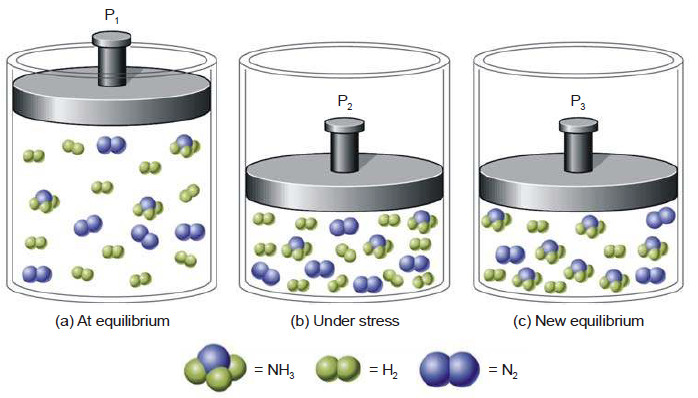Illustratation of Le Chatelier’s principle. (a) System at equilibrium with 10H2 , 5N2 , and 3NH3 , for a total of 18 molecules. (b) The same molecules are forced into a smaller volume, creating a stress on the system. (c) Six H2 and 2N2 have been converted to 4NH3. A new equilibrium has been established with 4H2 , 3N2 , and 7NH3 , a total of 14 molecules. The stress is partially relieved by the reduction in the total number of molecules.

### Effect of change of concentration on Ammonia Synthesis reaction

– Let us illustrate the effect of change of concentration on a system at equilibrium by taking example of the ammonia synthesis reaction:

N2(g) + 3H2(g) ↔ 2NH3(g)

– When N2 (or H2) is added to the equilibrium already in existence (equilibrium I), the equilibrium will shift to the right so as to reduce the concentration of N2 (Le Chatelier’s principle).

– The concentration of NH3 at the equilibrium II is more than at equilibrium I.

– The results in a particular case after the addition of one mole/litre are given below.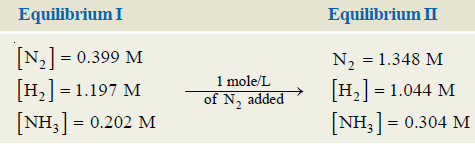– Obviously, the addition of N2 (a reactant) increases the concentration of NH3, while the
concentration of H2 decreases.

– Thus to have a better yield of NH3, one of the reactants should be added in excess.

– A change in the concentration of a reactant or product can be effected by the addition or removal of that species. Let us consider a general reaction:

A + B ↔ C

– When a reactant, say, A is added at equilibrium, its concentration is increased. The forward reaction alone occurs momentarily.

– According to Le Chatelier’s principle, a new equilibrium will be established so as to reduce the concentration of A.

– Thus the addition of A causes the equilibrium to shift to right. This increases the concentration (yield) of the product C.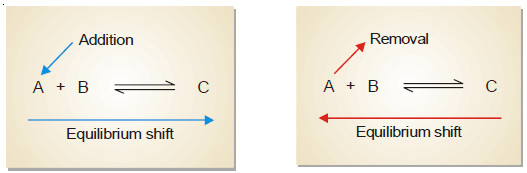– Following the same line of argument, a decrease in the concentration of A by its removal from the equilibrium mixture, will be undone by shift to the equilibrium position to the left. This reduces the concentration (yield) of the product C.

## Effect of a change of pressure According to Le Chatelier’s principle

– To predict the effect of a change of pressure, Le Chatelier’s principle may be stated as:

when pressure is increased on a gaseous equilibrium reaction, the equilibrium will shift in a direction which tends to decrease the pressure.

– The pressure of a gaseous reaction at equilibrium is determined by the total number of molecules it contains.

– If the forward reaction proceeds by the reduction of molecules, it will be accompanied by a decrease of pressure of the system and vice versa.

– Let us consider a reaction,

A + B ↔ C

– The combination of A and B produces a decrease of number of molecules while the decomposition of C into A and B results in the increase of molecules.

– Therefore, by the increase of pressure on the equilibrium it will shift to right and give more C. A decrease in pressure will cause the opposite effect.

– The equilibrium will shift to the left when C will decompose to form more of A and B.

– The reactions in which the number of product molecules is equal to the number of reactant molecules,

e.g.,        H2(g) + I2(g) ↔ 2HI

are unaffected by pressure changes. In such a case the system is unable to undo the increase or decrease of pressure.

– In light of the above discussion, we can state a general rule to predict the effect of pressure changes on chemical equilibria.

– The increase of pressure on a chemical equilibrium shifts it in that direction in which the number of molecules decreases and vice-versa.

– This rule is illustrated by the examples listed in this Table.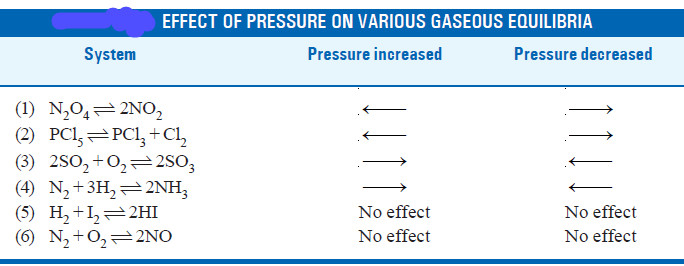## Effect of a change of temperature According to Le Chatelier’s principle

– Chemical reactions consist of two opposing reactions.

– If the forward reaction proceeds by the evolution of heat (exothermic), the reverse reaction occurs by the absorption of heat (endothermic).

– Both these reactions take place at the same time and equilibrium exists between the two.

– If temperature of a reaction is raised, heat is added to the system. The equilibrium shifts in a direction in which heat is absorbed in an attempt to lower the temperature.

– Thus the effect of temperature on an equilibrium reaction can be easily predicted by the following version of the Le Chatelier’s principle.

When temperature of a reaction is increased, the equilibrium shifts in a direction in which heat is absorbed.

– Let us consider an exothermic reaction

A + B ↔ C + heat

– When the temperature of the system is increased, heat is supplied to it from outside.

– According to Le Chatelier’s principle, the equilibrium will shift to the left which involves the absorption of heat.

– This would result in the increase of the concentration of the reactants A and B.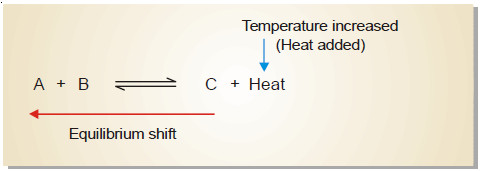– In an endothermic reaction:

X + Y + heat ↔ Z

– The increase of temperature will shift the equilibrium to the right as it involves the absorption of heat.

– This increases the concentration of the product Z.

– In general, we can say that the increase of temperature favours the reverse change in an exothermic reaction and the forward change in an endothermic reaction.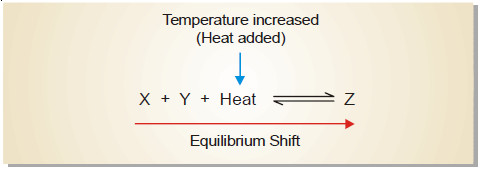### Formation of Ammonia from N2 and H2

– The synthesis of ammonia from nitrogen and hydrogen is an exothermic reaction.

N2 + 3H2 ↔ 2NH3 + 22.2 kcal

– When the temperature of the system is raised, the equilibrium will shift from right-to-left which
absorbs heat (Le Chatelier’s principle) This results in the lower yield of ammonia.

– On the other hand, by lowering the temperature of the system, the equilibrium will shift to the right which evolves heat in an attempt to raise the temperature.

– This would increase the yield of ammonia. But with decreasing temperature, the rate of reaction is slowed down considerably and the equilibrium is reached slowly.

– Thus in the commercial production of ammonia, it is not feasible to use temperature much lower than 500°C.

– At lower temperature, even in the presence of a catalyst, the reaction proceeds too slowly to be practical.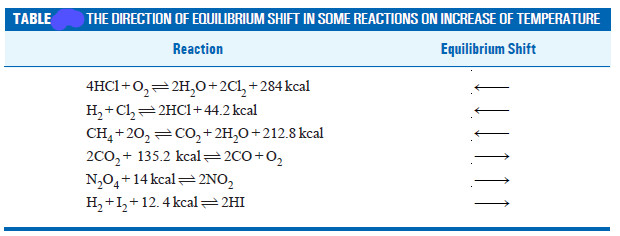## Conditions for maximum yield in industrial processes

– With the help of Le Chatelier’s principle we can work out the optimum conditions for securing
the maximum yield of products in industrial processes.

### Synthesis of Ammonia (Haber Process)

– The manufacture of ammonia by Haber process is represented by the equation

N2(g) + 3H2(g) ↔ 2NH3(g) + 22.0 kcal

– A look at the equation provides the following information:

(a) the reaction is exothermic

(b) the reaction proceeds with a decrease in the number of moles.

(1) Low temperature

– By applying Le Chatelier’s principle, low temperature will shift the equilibrium to the right.  This gives greater yield of ammonia.

– In actual practice a temperature of about 450°C is used when the percentage of ammonia in the equilibrium mixture is 15.

(2) High pressure

– High pressure on the reaction at equilibrium favours the shift of the equilibrium to the right.

– This is so because the forward reaction proceeds with a decrease in the number of moles.

– A pressure of about 200 atmospheres is applied in practice.

(3) Catalyst

– As already stated, low temperature is necessary for higher yield of ammonia. But at relatively low temperatures, the rate of reaction is slow and the equilibrium is attained in a long time.

– To increase the rate of reaction and thus quicken the attainment of equilibrium, a catalyst is used.

– Finely divided iron containing molybdenum is employed in actual practice.

– Molybdenum acts as a promoter that increases the life and efficiency of the catalyst. Pure N2 and H2 gases are used in the process.

– Any impurities in the gases would poison the catalyst and decrease its efficiency.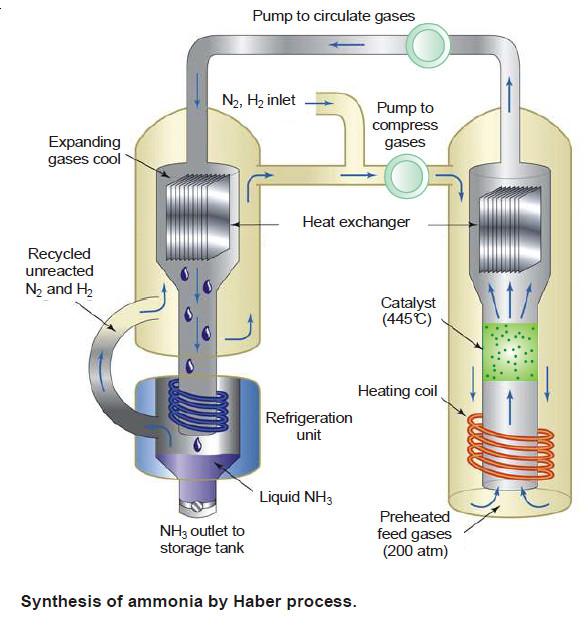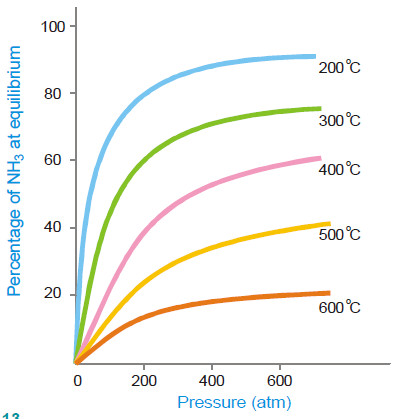Effect of temperature and pressure on the equilibrium N2 + 3H2↔ 2NH3 Increasing temperature decreases the percentage of NH at equilibrium. Increasing pressure increases the percentage of NH3 at equilibrium

### Manufacture of Sulphuric acid (Contact Process)

– The chief reaction used in the process is:

2SO2(g) + O2(g) ↔ 2SO3(g) + 42 kcal

– Following information is revealed by the above equation:

(a) the reaction is exothermic.

(b) the reaction proceeds with a decrease in number of moles.

– On the basis of Le Chatelier’s principle, the conditions for the maximum yield can be worked out as below:

(1) Low temperature

– Since the forward reaction is exothermic, the equilibrium will shift on the right at low temperature.

– An optimum temperature between 400-450°C is required for the maximum yield of sulphur trioxide.

(2) High pressure

– Since the number of moles are decreased in the forward reaction, increase of pressure will shift the equilibrium to the right.

– Thus for maximum yield of SO3, 2 to 3 atmosphere pressure is used.

(3) Catalyst

– At the low temperature used in the reaction, the rate of reaction is slow and the equilibrium is attained slowly.

– A catalyst is, therefore, used to speed up the establishment of the equilibrium.

– Vanadium pentoxide, V2O5, is commonly used and it has replaced the earlier catalyst platinum asbestos which was easily poisoned by the impurities present in the reacting gases.

– All the same, SO2 and O2 used for the manufacture of sulphuric acid must be pure and dry.

### Manufacture of Nitric acid (Birkeland-Eyde process)

Nitric acid is prepared on a large scale by making use of the reaction:

N2(g) + O2(g) ↔ 2NO(g) – 43.2 kcal

– The equation tells us that:

(a) the reaction proceeds with no change in the number of moles.

(b) the reaction is endothermic and proceeds by absorption of heat.

– The favourable conditions for the maximum yield of NO are:

(1) High temperature.

– Since the forward reaction is endothermic, increase of temperature will favour it (Le Chatelier’s principle).

– Thus a high temperature of the order of 3000°C is employed to get high yield of nitric acid.

(2) No effect of pressure.

– Since the forward reaction involves no change in the number of moles, a change in pressure has no effect on the equilibrium.

(3) High concentration.

– The formation of nitric oxide is favoured by using high concentrations of the reactants i.e. N2 and O2.